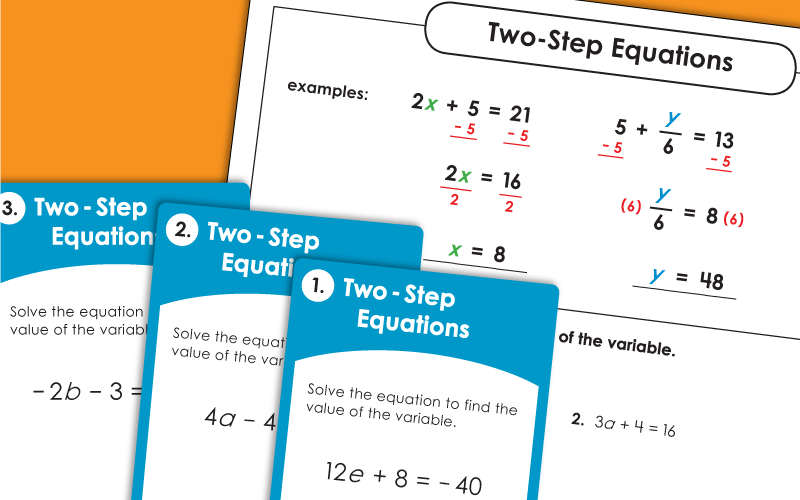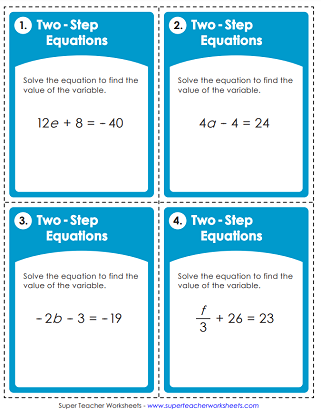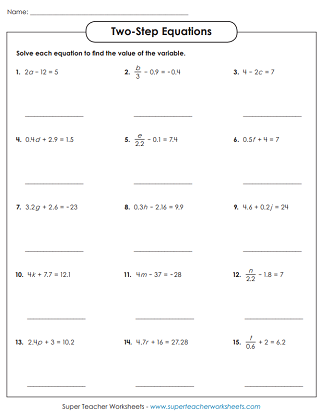# Algebraic Equations (Two-Step)

Practice solving two-step algebraic equations with these printable worksheets and task cards. Common Core Standard: 7.EE.B.4## Level: BasicPositive Whole Numbers

This worksheet has 15 two-step equations for students to solve. There is a model problem at the top. These basic level problems do not include exponents or parenthesis.
Use addition, subtraction, and multiplications to determine the value of x in each equation.

## Intermediate Level:Positive & Negative Integers

Find the value of the variable for each two-step equation. The intermediate level contains problems with negative and positive integers.
This worksheet has 15 equations for students to solve and find the values of the variables.
This file contains 30 task cards with 30 different equations. Students must isolate the variable using two steps to solve.

## Advanced Level:Decimals, Exponents, & Parenthesis

Give this worksheet to students ready to try their hand at more advanced two-step equations including decimals.
One-Step Equations

If two-step equations are too advanced, go down a level and use these single-step equation resources.

More Algebra Topics

We have a variety of algebra and pre-algebra topics for teaching students about independent/dependent variables, expressions, equations, and inequalities.

## Sample Worksheet ImagesMy Account
Site Information